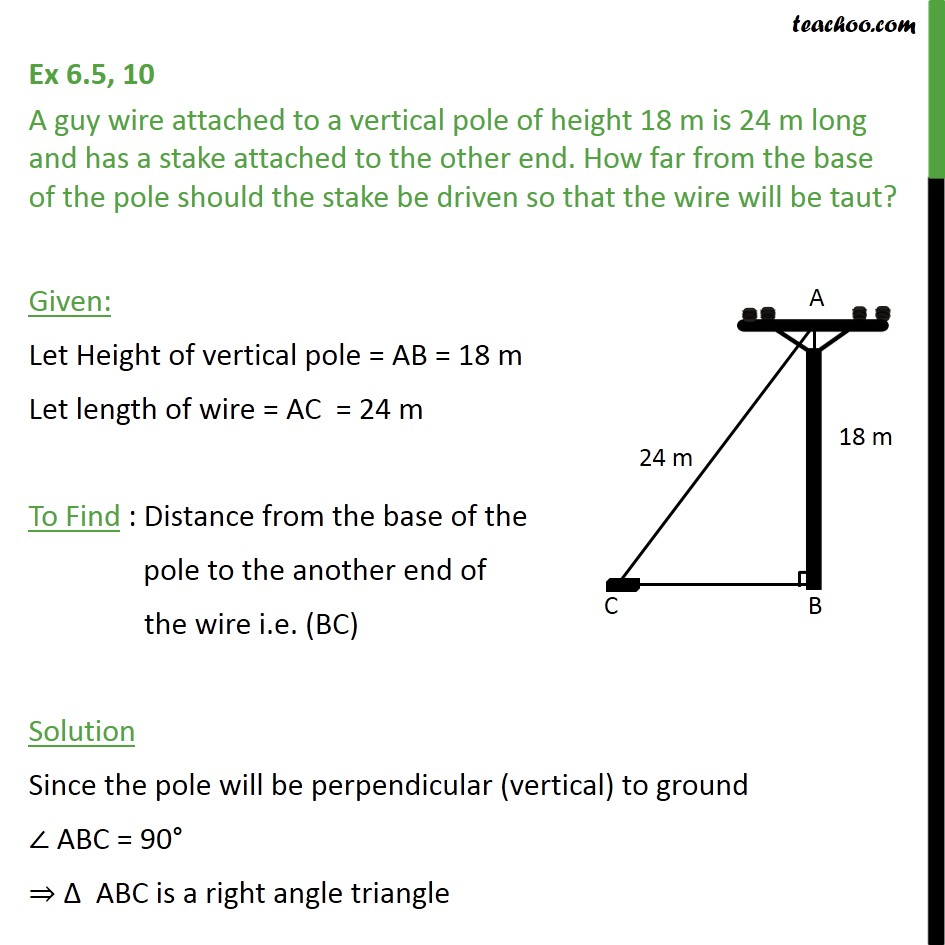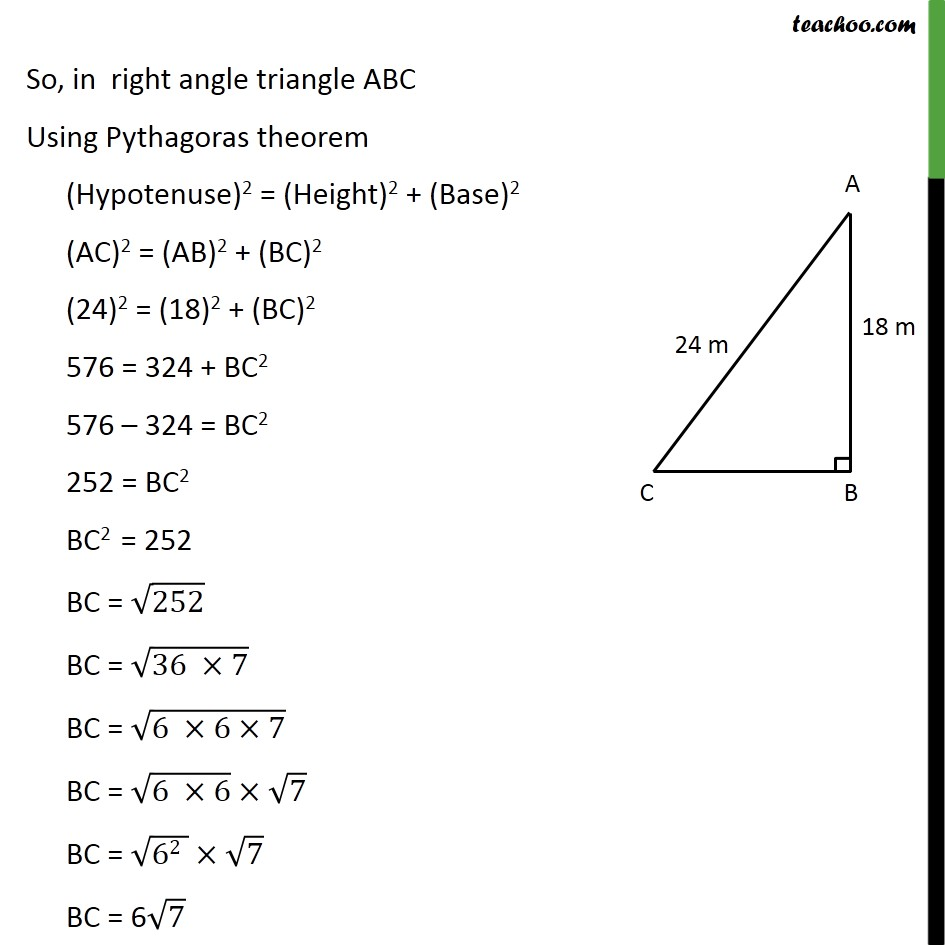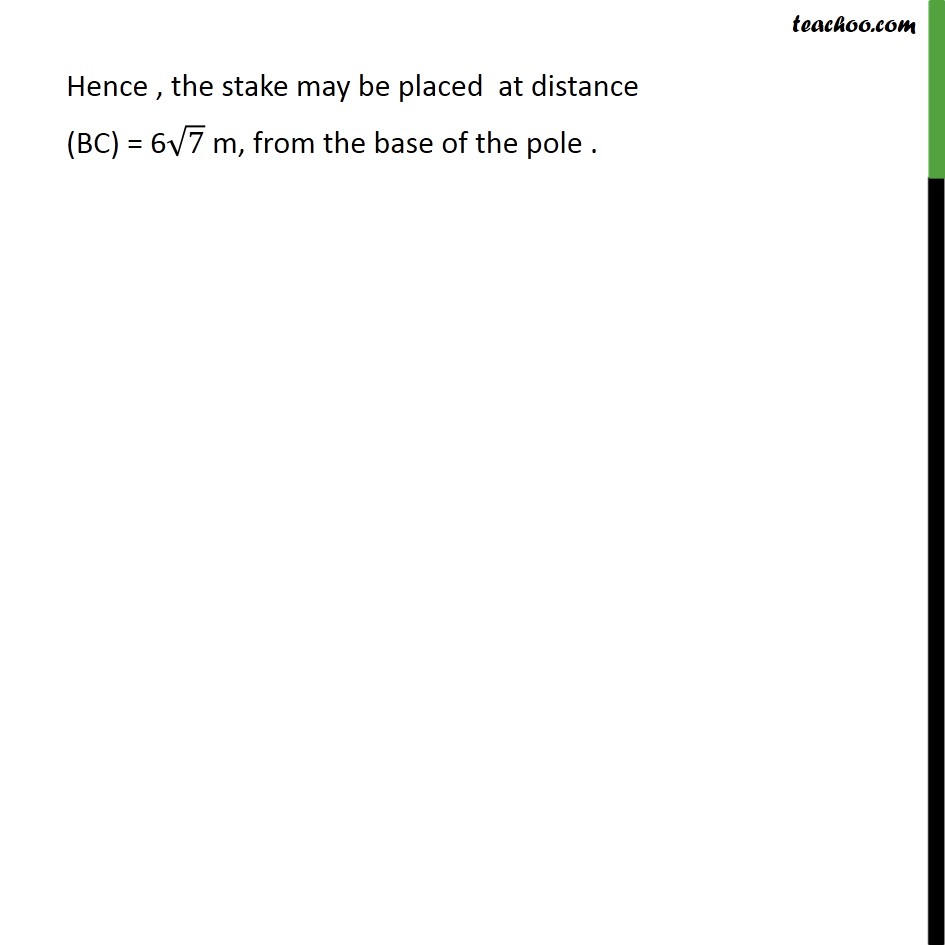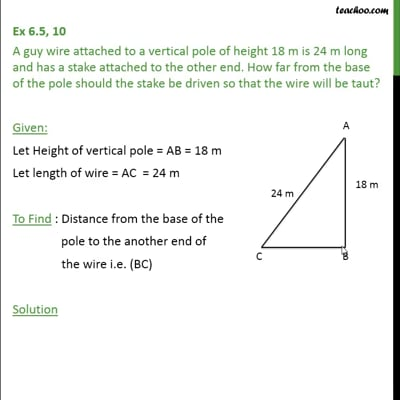Ex 6.5

Chapter 6 Class 10 Triangles (Term 1)
Serial order wiseThis video is only available for Teachoo black users

### Transcript

Ex 6.5, 10 A guy wire attached to a vertical pole of height 18 m is 24 m long and has a stake attached to the other end. How far from the base of the pole should the stake be driven so that the wire will be taut? Given: Let Height of vertical pole = AB = 18 m Let length of wire = AC = 24 m To Find : Distance from the base of the pole to the another end of the wire i.e. (BC) Solution Since the pole will be perpendicular (vertical) to ground ABC = 90 ABC is a right angle triangle So, in right angle triangle ABC Using Pythagoras theorem (Hypotenuse)2 = (Height)2 + (Base)2 (AC)2 = (AB)2 + (BC)2 (24)2 = (18)2 + (BC)2 576 = 324 + BC2 576 324 = BC2 252 = BC2 BC2 = 252 BC = 252 BC = (36 7) BC = (6 6 7) BC = (6 6) 7 BC = (62 ) 7 BC = 6 7 Hence , the stake may be placed at distance (BC) = 6 7 m, from the base of the pole .